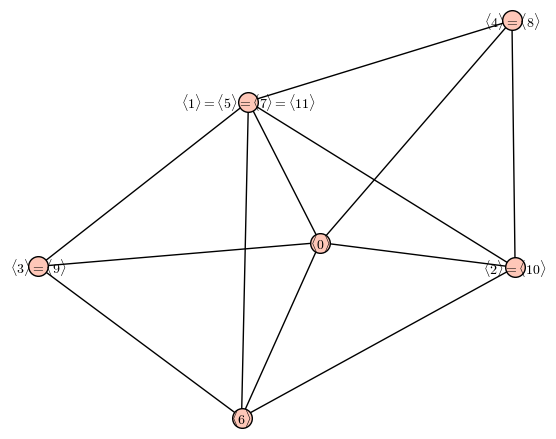# How to draw the following graph

I want to write the following code in sagemath but unable to write it:

Suppose we consider the group $\Bbb Z_n$.

We consider an element $a\in \Bbb Z_n$ and form the subgroup generated by $a$ i.e. $\langle a\rangle ={a,2a,3a,\ldots 0}.$

We form a graph $G$ whose vertices are $\langle a\rangle$ and $\langle a\rangle$ and $\langle b\rangle$ are adjacent if either $\langle a\rangle \subset \langle b\rangle$ or $\langle b\rangle \subset \langle a\rangle$ .

How to plot the graph $G$ is Sagemath?

I am giving an example to clear the question:

Consider $\Bbb Z_4$ then consider $\langle 0\rangle$, $\langle 1\rangle$ , $\langle 2\rangle$, $\langle 3\rangle$

Clearly $\langle 0\rangle ={0}$, $\langle 1\rangle={1,2,3,0}$ , $\langle 2={2,0}\rangle$, $\langle 3={0,1,2,3}\rangle$.

Thus the graph $G$ has vertices as $\langle 0\rangle$, $\langle 1\rangle$ , $\langle 2\rangle$, $\langle 3\rangle$ and $\langle 0\rangle$ is adjacent to $\langle 1\rangle$ , $\langle 2\rangle$, $\langle 3\rangle$,

$\langle 1\rangle$ is adjacent to $\langle 2\rangle$ , $\langle 0\rangle$,

$\langle 2\rangle$ is adjacent to $\langle 1\rangle$ , $\langle 0\rangle$, $\langle 3\rangle$

and $\langle 3\rangle$ is adjacent to $\langle 0\rangle$, $\langle 2\rangle$

Thus $G$ becomes

https://imgur.com/Ef9P4uz

edit retag close merge delete

1

Why are $1$ and $3$ different vertices in your graph? They generate the same additive subgroup, i.e. $\langle 1\rangle = \langle 3 \rangle$.

Regarding the picture, i would say that @Captcha means that the vertices are elements of $\mathbb{Z}_n$, not its subgroups

Sort by » oldest newest most voted

Unfortunately, if you start defining your groups the "normal" way with groups.misc.AdditiveCyclic, you will not find a way to construct subgroups (at least i did not find any subgroup method or similar). So, you will have to use permuation groups (they can compute subgroups) wich is already a workaround.

So, let me suggest to work directly with this basic property of cyclic groups:

$\langle i \rangle \subseteq \langle j \rangle$ in $\mathbb{Z}_n$ if, and only if, $gcd(j,n) | gcd(i,n)$ (where $|$ stands for "divides").

Hence, the following code should do the trick:

def gr(n):
G = Graph(n)
for i in range(n):
for j in range(i+1,n):
a,b = gcd(i,n), gcd(j,n)
if (a.divides(b) or b.divides(a)) and a != b:
return G


Then, you can do:

gr(4).plot()

more

I am extremely thankful for the answer. Can you kindly say how to start learning coding in sagemath? How to know the way to write loops , how to draw a graph, add edges as you have etc.??

1

I think you should first learn some python, for loops, functions, tests, etc. There are tons on nice tutorials on the web. Then, you will learn Sage specifics from the experience, you can have a look at the thematic tutorials at : https://doc.sagemath.org/html/en/them...Here's a way:

n = 12
from collections import defaultdict
subgroup_gens = defaultdict(list)
for g in G:
subgroup_gens[G.submodule([g])].append(ZZ(g))
mylabel = lambda H: LatexExpr('$' + '='.join('\\langle ' + str(g) + ' \\rangle' for g in subgroup_gens[H]) + '$')
Graph([(mylabel(H),mylabel(K)) for H in subgroup_gens.keys() for K in subgroup_gens.keys() if H != K and H.is_submodule(K)]).plot(figsize=6)more